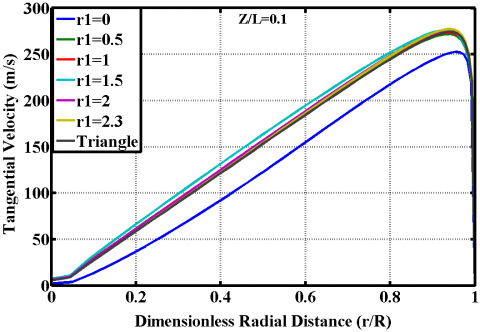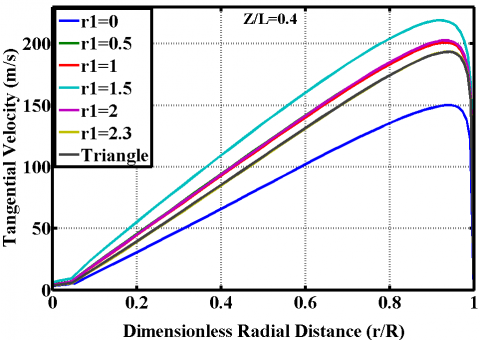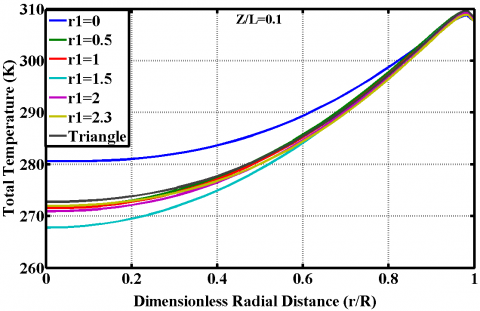# 3D CFD analysis of heat transfer process inside vortex chamber of Ranque-Hilsch vortex tube- optimization of bottom radius

3D CFD analysis of heat transfer process inside vortex chamber of Ranque-Hilsch vortex tube- optimization of bottom radius

Department of Mathematics, Payame Noor University (PNU), Tehran 19395-3697, Iran

Department of Mechanical and Industrial Engineering, Ryerson University, 350 Victoria St., Toronto, Ontario, Canada

Corresponding Author Email:
Page:
5-10
|
DOI:
https://doi.org/10.18280/psees.020102
10 April 2018
| |
Accepted:
12 April 2018
| | Citation

OPEN ACCESS

Abstract:

This research describes numerical investigations on the impacts of inner radius (bottom r1) on the thermal capability (heating and cooling) of the vortex tube and the heat and mass transfer between the cold and hot vortex cores inside a Ranque-Hilsch vortex tube applying the 3D CFD models. The cooling and heating capabilities increase severely with an increase in r1 value upto 1.5 mm (optimum value) and beyond this optimal value the thermal effectiveness of vortex tube decreases. The results have been compared to the experimental values with good agreement (less than 7 %).

Keywords:

vortex tube, inner radiuses, heat transfer, energy separation

1. Introduction

## 1.pngFigure 1. A schematic drawing of Ranque-Hilsch vortex tube

2. Basic Concepts

The performance measurements on the VT systems (usually) are pointed and presented based on the temperature differences (there is no difference what kind of the VT is used, RHVT, PVT). There are three definitions; first, the cold temperature difference or $\Delta T_{\text { cold }}$ (difference between cold and inlet sides), the total temperature difference or $\Delta T$ (difference between cold and hot sides) and the hot temperature difference or $\Delta T_{\text { hot }}$ (difference between hot and inlet sides), these definitions are as bellow:

$\Delta T_{h}=T_{h}-T_{\text {inlet}}$    (1)

$\Delta T_{C}=T_{i n l e t}-T_{C}$    (2)

3. Physical Model Description

The 3D CFD model is created on basis of that was used by Skye et al.  in their experimental work. It is noteworthy that, an ExairTM 708 slpm vortex tube was used by Skye et al.  to perform all tests and to take all of the experimental data. Figure. 2 shows the geometrical parameters of the vortex tube which is under optimization. The 3D CFD mesh grid is shown in Figure. 3. In this model a regular organized mesh grid has been used. All radial line of this model of meshing has been connected to the centerline and the circuit lines have been designed organized from wall to centerline. So, the volume units that have been created in this model are regular cubic volumes. This meshing system helps the computations to be operated faster than the irregular and unorganized meshing, and the procedure of computations have been done more precisely.

## 2.pngFigure 2. Schematic of vortex tube

For this reason the CFD model has been assumed a rotational periodic condition. Hence, only a sector of the flow domain with angle of 60° needs to consider for computations as shown in Figure 3.

## 3.pngFigure 3. 3D CFD model of vortex chamber

4. Results and Discussion

The numerical results achieved from the model, which involve the effect of edge radius change on different vortex tube parameters, are presented in this section. Although the separation at both exits is affected by the change in the edge radius inside the vortex-chamber, it is the cold exit temperature separation that is of special interest to us, owing to the potential industrial applications for gas flows of such low temperatures. In order to demonstrate the effect of edge radius change on radial profiles of tangential velocity, the profiles at three axial locations (Z/L= 0.1, 0.4, and 0.7) at the cold mass fraction 0.3 were analyzed, the diagrams of which are depicted in Figure.4. As seen in these Figureures, a vortex-chamber with a radius edge creates a larger magnitude of tangential velocity in comparison with a vortex-chamber without a radius edge in the critical zone. The diagrams show that at Z/L= 0.1 (near the chamber), the magnitude of tangential velocity at r1=2, 2.3 and 1.5 is greater than its value at r1=0, 0.5, 1 and triangle model.

## 4-1.png## 4-2.png## 4-3.pngFigure 4. Radial profile of tangential velocity at Z/L=0.1, Z/L=0.4 and 0.7 for ε =0.3

In Figureure 4, the radial profiles for the tangential velocity at Z/L= 0.1, 0.4, and 0.7 are provided for all of the created models. The swirl (tangential) velocity magnitude decreases as we move towards the hot end exit. This is clearly observable that the tangential velocity magnitude in the model of r1=1.5 is the greatest swirl velocity among the all of created models. The radial profile of the tangential velocity shows a free vortex near the wall. On the other hand, another, forced, vortex is formed in the core, in which swirl velocity values decrease as we move towards the centerline of working tube.

The total temperature variations for different edge radiuses at the three axial locations Z/L= 0.1, 0.4, and 0.7 are presented in Figure 5.

## 5-1.png## 5-2.png## 5-3.pngFigure 5. Radial profile of total temperature at Z/L=0.1, Z/L=0.4 and 0.7 for ε =0.3

For all the edge radiuses, the maximum total temperature occurred near the periphery of the tube wall. The low temperature zone in the core coincides with the negligible tangential velocity zone. At the all of axial locations, the centerline total temperature in case (c1) has the lowest magnitude among the created models. This means that; a vortex tube which have a vortex-chamber with the edge radius r1=1.5 can create a colder stream than common produced models. As shown in temperature Figureures, the common model of vortex chamber (r1=0) is not an efficient model. Figureure 6 show the total pressure variations for different edge radiuses at the three axial locations Z/L= 0.1, 0.4, and 0.7 as a function of dimensionless radial distance of working tube. The maximum total pressure is observed near the periphery of the tube wall.

## 6-1.png## 6-2.png## 6-3.pngFigure 6. Radial profile of total pressure at Z/L=0.1, Z/L=0.4 and 0.7 for ε =0.3

As seen in Figure. 6, fluid stream has greater total pressure in case (c1) in comparison with other cases. Figureure 6 demonstrates that the case (c1) has better swirl velocity, total pressure and the efficient total temperature than other cases. Case (c1) has lower cold exit temperature than the other models. So case (c1) is the efficient case. Figure. 7 shows the total temperature contour of optimal model.

## 7-1.png## 7-2.png## 7-3.png## 7-4.pngFigure 7. Total temperature contour of optimized model

5. Conclusions

An optimization model of vortex-chamber has been modeled in this paper. We developed a 3D computational fluid dynamic model to simulate a vortex-chamber of vortex tube. Some changes have been implemented on vortex-chamber’s geometry and the effect of these changes has been studied to achieve the better performance of vortex tube. Primitive results have been validated with Skye et al.’s experimental data and show a good agreement with these results. The existence of edges at point 1 and 2 inside the vortex-chamber is an effective parameter of vortex tube’s performance. Optimized value of r1 at point 1 has been determined and that is 1.5 mm.

References

 Ranque GJ. (1933). Experiments on expansion in a vortex with simultaneous exhaust of hot air and cold air. Le J. de Physique et le Radium 4: 112-114.

 Hilsch R. (1947). The use of expansion of gases in a centrifugal field as a cooling process. Rev Sci Instrum. 18: 108-113. https://doi.org/10.1063/1.1740893

 Dutta T, Sinhamahapatra KP, Bandyopadhyay SS. (2011). Numerical investigation of gas species and energy separation in the Ranque–Hilsch vortex tube using real gas model. Int. J. Refrigeration 26(8): 2118-2128.

 Mohammadi S, Farhadi F. (2013). Experimental analysis of a Ranque–Hilsch vortex tube for optimizing nozzle numbers and diameter. Applied Thermal Engineering 61(2): 500-506.

 Rafiee SE, Sadeghiazad MM. (2016). Heat and mass transfer between cold and hot vortex cor,es inside ranque-hilsch vortex tube-optimization of hot tube length. International Journal of Heat and Technology 34(1): 31-38. https://doi.org/10.18280/ijht.340105

 Baghdad M, Ouadha A, Imine O, Addad Y. (2011). Numerical study of energy separation in a vortex tube with different RANS models. Int. J. Thermal Sciences 50(12): 2377-2385. https://doi.org/10.1016/j.ijthermalsci.2011.07.011

 Rafiee SE, Sadeghiazad MM. (2016). Three-dimensional computational prediction of vortex separation phenomenon inside Ranque-Hilsch vortex tube. Aviation 20(1): 21-31. https://doi.org/10.3846/16487788.2016.1139814

 Rafiee SE, Rahimi M. (2013). Experimental study and three-dimensional (3D) computational fluid dynamics (CFD) analysis on the effect of the convergence ratio, pressure inlet and number of nozzle intake on vortex tube performance-Validation and CFD optimization. Energy 63: 195-204. https://doi.org/10.1016/j.energy.2013.09.060

 Pourmahmoud N, Abbaszadeh M, Rashidzadeh M. (2016). Numerical simulation of effect of shell heat Transfer on the vortex tube performance. International Journal of Heat and Technology 34(2): 293-301. https://doi.org/10.18280/ijht.340220.

 Pourmahmoud N, Esmaily R, Hassanzadeh A. (2015). CFD investigation of vortex tube length effect as a designing criterion. International Journal of Heat and Technology 33(1): 129-136. https://doi.org/10.18280/ijht.330118

 Rafiee SE, Ayenehpour S, Sadeghiazad MM. (2016). A study on the optimization of the angle of curvature for a Ranque–Hilsch vortex tube, using both experimental and full Reynolds stress turbulence numerical modeling. Heat and Mass Transfer 52(2): 337-350.

 Rahimi M, Rafiee SE, Pourmahmoud N. (2013). Numerical investigation of the effect of divergent hot tube on the energy separation in a vortex tube. International Journal of Heat and Technology 31(2): 17-26.

 Rafiee SE, Rahimi M, Pourmahmoud N. (2013). Three-Dimensional numerical investigation on a commercial vortex tube based on an experimental model- part I: optimization of the working tube radius. International Journal of Heat and Technology 31(1): 49-56.

 Rafiee SE, Sadeghiazad MM. (2015). 3D numerical analysis on the effect of rounding off edge radius on thermal separation inside a vortex tube. International Journal of Heat and Technology 33(1): 83-90.

 Pourmahmoud N, Hasanzadeh A, Rafiee SE, Rahimi M. (2012). Three-dimensional numerical investigation of effect of convergent nozzles on the energy separation in a vortex tube. International Journal of Heat and Technology 30(2): 133-140.

 Pourmahmoud N, Rafiee SE, Rahimi M, Hasanzadeh A. (2013). Numerical energy separation analysis on the commercial Ranque-Hilsch vortex tube on basis of application of different gases. Scientia Iranica 20(5): 1528-1537.

 Rafiee SE, Sadeghiazad MM. (2016). Three-dimensional CFD simulation of fluid flow inside a vortex tube on basis of an experimental model- the optimization of vortex chamber radius. International Journal of Heat and Technology 34(2): 236-244. https://doi.org/10.18280/ijht.340212

 Rafiee SE, Sadeghiazad MM. (2016). Three-dimensional numerical investigation of the separation process inside vortex tube using different operating conditions. Journal of Marine Science and Application 06: 1-10. https://doi.org/10.1007/s11804-016-1348-8

 Rafiee SE, Sadeghiazad MM. (2014). Effect of conical. valve angle on cold-exit temperature of vortex tube. Journal of Thermophysics and heat transfer 28: 785-794. https://doi.org/10.2514/1.T4376

 Rafiee SE, Sadeghiazad MM. (2014). Three-dimensional and experimental investigation on the effect of cone length of throttle valve on thermal performance of a vortex tube using k–ɛturbulence model. Applied Thermal Engineering, 66(1–2): 65–74. https://doi.org/10.1016/j.applthermaleng.2014.01.073

 Rafiee SE, Sadeghiazad MM, Mostafavinia N. (2015). Experimental and numerical investigation on effect of convergent angle and cold orifice diameter on thermal performance of convergent vortex tube. J. Thermal Sci. Eng. Appl. 7(4). https://doi.org/10.1115/1.4030639

 Rafiee SE, Sadeghiazad MM. (2017). Efficiency evaluation of vortex tube cyclone separator. Applied Thermal Engineering 114(5): 300–327. https://doi.org/10.1016/j.applthermaleng.

 Rafiee SE, Sadeghiazad MM. (2017). Experimental and 3D-CFD investigation on optimization of the air separator structural parameters for maximum separation efficiency. Separation Science and Technology 52(5): 903-929. https://doi.org/10.1080/01496395.2016.1267755

 Rafiee SE, Sadeghiazad M.M. (2016). Experimental study and 3D CFD analysis on the optimization of throttle angle for a convergent vortex tube. Journal of Marine Science and Application 15(4): 388–404. https://doi.org/10.1007/s11804-016-1387-1

 Rafiee SE, Sadeghiazad MM. (2016). Experimental and 3D-CFD study on optimization of control valve diameter for a convergent vortex tube. Frontiers in Heat and Mass Transfer 7(1): 1-15. https://doi.org/10.5098/hmt.7.13

 Rafiee SE., Sadeghiazad MM. (2016). Experimental and 3D CFD investigation on energy separation inside a convergent vortex tube air separator. Scientia Iranica 23(4).

 Rafiee SE, Sadeghiazad MM. (2014).3D CFD exergy analysis of the performance of a counter flow vortex tube, International Journal of Heat and Technology 32(1-2): 71-77.

 Rafiee SE, Rahimi M. (2014). Three-dimensional simulation of fluid flow and energy separation inside a vortex tube. Journal of Thermophysics and Heat Transfer 28: 87-99. https://doi.org/10.2514/1.T4198

 Lorenzini E, Spiga M. (1982). Aspetti fluidodinamici della separazione isotopica mediante tubi a vortice di Hilsch Ingegneria 121-126 (maggio-giugno).

 Rafiee SE, Sadeghiazad MM. (2017). Experimental and 3D CFD investigation on heat transfer and energy separation inside a counter flow vortex tube using different shapes of hot control valves. Applied Thermal Engineering 110: 648-664.

 Pourmahmoud N, Rahimi M, Rafiee SE, Hassanzadeh A, (2014) A numerical simulation of the effect of inlet gas temperature on the energy separation in a vortex tube. Journal of Engineering Science and Technology 9(1): 81-96.

 Mirandola A, Lorenzini E. (2016). Energy, environment and climate: from the past to the future. International Journal of Heat and Technology 34(2): 159-164. https://doi.org/10.18280/ijht.340201

 Skye HM, Nellis GF, Klein SA. (2006). Comparison of CFD analysis to empirical data in a commercial vortex tube. Int. J. Refrig 29: 7180. https://doi.org/10.1016/j.ijrefrig.2005.